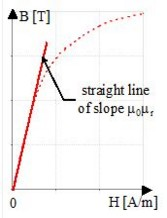# Linear approximation * exponential function of T

## Presentation

This model defines a B(H) behavior law:

• linear (without taking the saturation into consideration)
• for an isotropic material
• through a relative permeability μr which decreases in an exponential way when the temperature increases

It can be used for low magnetic fields as long as saturation is not reached.

## Mathematical model

This model is a straight line whose μr slope varies with the temperature.

The corresponding mathematical formula is written:

B(H,T)= μ0H+ μ0( μr0 - 1)H.COEF(T)

where:

• μ0 is the permeability of vacuum; μ0 = 4 π 10-7 [H/m]
• μr0 is the relative permeability of the material for T = 0°C
• COEF(T) is the previously described temperature coefficient

The shape of B(H) curve is presented in the figure below.